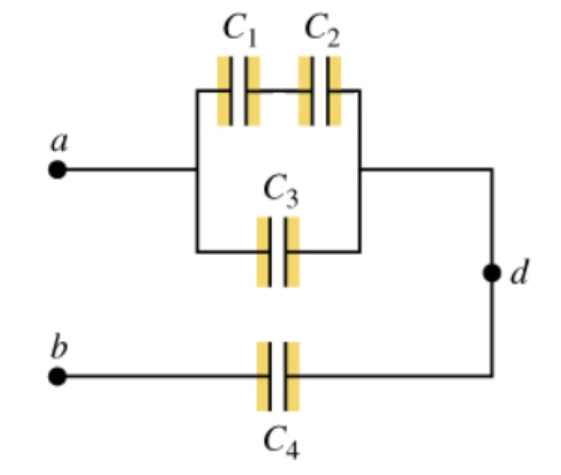# Problem: In the figure (Figure 1), each capacitor has 4.30 μF and Vab = 32.0 VPart A: Calculate the charge on capacitor C1.Part B: Calculate the potential difference across capacitor C1Part C: Calculate the charge on capacitor C2.Part D: Calculate the potential difference across capacitor C2.Part E: Calculate the charge on capacitor C3.Part F: Calculate the potential difference across capacitor C3.Part G: Calculate the charge on capacitor C4.Part H: Calculate the potential difference across capacitor C4Part I: Calculate the potential difference between points a and d

###### FREE Expert Solution

For capacitors in series, the equivalent capacitance is:

$\overline{)\frac{\mathbf{1}}{{\mathbf{C}}_{\mathbf{e}\mathbf{q}}}{\mathbf{=}}\frac{\mathbf{1}}{{\mathbf{C}}_{\mathbf{1}}}{\mathbf{+}}\frac{\mathbf{1}}{{\mathbf{C}}_{\mathbf{2}}}{\mathbf{+}}{\mathbf{.}}{\mathbf{.}}{\mathbf{.}}{\mathbf{+}}\frac{\mathbf{1}}{{\mathbf{C}}_{\mathbf{n}}}}$

For capacitors in parallel, the equivalent capacitance is:

$\overline{){{\mathbf{C}}}_{\mathbf{e}\mathbf{q}}{\mathbf{=}}{{\mathbf{C}}}_{{\mathbf{1}}}{\mathbf{+}}{{\mathbf{C}}}_{{\mathbf{2}}}{\mathbf{+}}{\mathbf{.}}{\mathbf{.}}{\mathbf{.}}{\mathbf{+}}{{\mathbf{C}}}_{{\mathbf{n}}}}$

The charge stored in a capacitor:

$\overline{){\mathbf{Q}}{\mathbf{=}}{\mathbf{C}}{\mathbf{V}}}$

We also need to know that, parallel capacitors have the same potential difference across them. Series capacitors carry the same amount of charge.

C1 and C2 are in series:

C12 = (1/C1 + 1/C2)-1 = (1/4.30 + 1/4.30)-1 = 2.15 μF

C12 and C3 are in parallel:

C123 = C12 + C3 = 2.15 + 4.30 = 6.45 μF

C123 and C4 are in sereis.

99% (100 ratings)###### Problem Details

In the figure (Figure 1), each capacitor has 4.30 μF and Vab = 32.0 VPart A: Calculate the charge on capacitor C1.

Part B: Calculate the potential difference across capacitor C1

Part C: Calculate the charge on capacitor C2.

Part D: Calculate the potential difference across capacitor C2.

Part E: Calculate the charge on capacitor C3.

Part F: Calculate the potential difference across capacitor C3.

Part G: Calculate the charge on capacitor C4.

Part H: Calculate the potential difference across capacitor C4

Part I: Calculate the potential difference between points a and d